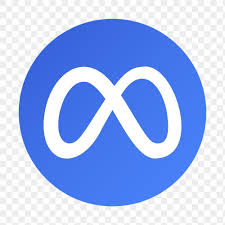## Longest Arithmetic Progression(This question has been seen in the interviews of the following companies: Facebook, Google, Microsoft)
In the array {1, 6, 3, 5, 9, 7}, the longest arithmetic sequence is 1, 3, 5, and 7

Dynamic programming with a map of a map.

``````

public static int llac(int[] array) {
if(array.length == 0) return 0;
int max = 1;
Map> index_to_llac = new HashMap<>(); //Map>
for(int current = 1; current < array.length; current++) {
index_to_llac.put(current, new HashMap<>());
for(int prev = 0; prev < current; prev++) {
int diff = array[current] - array[prev];
int length = 2;
if(index_to_llac.containsKey(prev) && index_to_llac.get(prev).containsKey(diff)) {
length = index_to_llac.get(prev).get(diff) + 1;
}
Map diff_to_llac = index_to_llac.get(current);
//if this is a arithmetic progression with [diff] exists before current, add 1 to the length to include current in the progression
//otherwise, [prev, current] forms a progression of size 2
diff_to_llac.put(diff, length);
max = Math.max(length, max);
}
}
return max;
}

``````